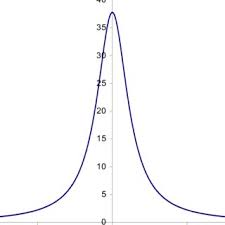## How to Calculate and Solve for Total Anomalous Mass | GravityThe image above represents total anomalous mass.

To compute for total anomalous mass, one essential parameter is needed and this parameter is Number of Area Segments (n).

The formula for calculating total anomalous mass:

ME = 23.9 Σ(ΔgδA)

Where:

ME = Total Anomalous Mass
Δg = Change in Gravity
δA = Area Segment

Let’s solve an example;
Find the total anomalous mass when the number of area of segments is 2 (change of gravity (Δg1) is 5, area segment (δA1) is 12, change of gravity (Δg2) is 8 and area segment (δA2) is 10).

This implies that;

δA1 = Area Segment = 12
Δg1 = Change in Gravity = 5
δA2 = Area Segment = 10
Δg2 = Change in Gravity = 8

Δg δA ΔgδA
5 12 60
8 10 80

Σ(ΔgδA) = 60 + 80
Σ(ΔgδA) = 140

Therefore,

ME = 23.9 Σ(ΔgδA)
ME = 23.9 (140)
ME = 3346

Therefore, the total anomalous mass is 3346 g.